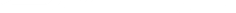### 5 Excel Functions for Aspiring Data Analysts

Excel is the most used data analysis tool in the world.

Due to the fact that you can do basic data entry, calculations, charting, advanced data analysis makes it the perfect choice in many small, medium, and large companies.

Excel functions are an integral part of doing calculations in Excel.

Here are my top five Excel functions that data analysts must know to get the most out of Excel.

• Excel Vlookup Function: Excel VLOOKUP Function allows you to quickly scan a huge list of items, identify where it is, and then fetch any value from that row. The V in VLOOKUP stands for Vertical as it would scan a vertical list of items. As data analysts, since we deal with copious amount of data, this function is indispensable.

• Excel If Function: If function has a simple task. Check for a given condition and return a specified value if the condition is TRUE, else return another value. If functions really help in taking decisions in cases, for example, where you need to know if the value is above threshold or not (such as in case of calculating commissions).  If you're a data analyst, or aspiring to be one, IF function will save you tons of time.

• Excel SUMIFS function: Often stakeholders/managers tweak their questions on the go. For example, your boss may ask you - "What were the sales of Product A in the west region?". And when you have the answer ready after doing all the calculation, he may suddenly tweak his question as ask - "And what about Product B in West region?". To handle such situations, learn the SUMIFs function as it allows you to easily change the conditions and get the answer (Note: for this above situations, Pivot Table works best).

• TEXT Functions: There are many text functions in Excel (such as LEFT, RIGHT, MID, LEN, and so on..). Data can come in all forms and shapes. If you get data that is in text format, you would need these functions to sail through. For example, suppose you get a combination of text and numbers (such as AERD2345), and you want to only get the numeric part of it. You need to extract the last four characters that you can do using the RIGHT function. Similarly, there can be many such situations where you need to use these Text functions.

• Excel SMALL/LARGE functions: A lot of times when you work with data, you need to keep a tab on the outliers. These could be values that are falling outside the acceptable range. Had there been only one or two of these, you could have handled it without a formula, but you sometime needs to have a look at lets say - bottom 10 values or top 10 values. In such cases, you would need the small/large functions in Excel. For example, In the small function, you can specify which number of smallest value you want. You can get the seconds smallest value, or third smallest value and so on.

If you're an aspiring analysts, start with these functions. You would definitely need to learn a lot more, but this can be a good starting point.

As I have been a data analyst myself, I also highly recommend learning Pivot Tables as it makes it super easy to crunch huge data sets and create quick summaries.

### Excel Functions Vs Excel Formulas?

Let's start with the very basics of learning of Excel functions.

Ever wondered what's the difference between an Excel function and an Excel formula?

A lot of people use these terms interchangeably. However, there is a big difference that you must know.

An Excel function is a formula that is already available in Excel. For example, if you want to add a set of numbers in Excel, you can use the SUM function in Excel. This function would have pre-defined syntax (input arguments in takes) and would give the result based on the inputs.

An Excel formula on the other side is something that is not available in Excel and you need to construct it. For example, suppose you have numbers in cell A1, A2, and A4, and you want to add these three cells, then you can also use =A1+A2+A4. This would be an Excel formula as it is something you have constructed.Now you could have also used the SUM function in this case, but when you create something that is not an inbuilt function in Excel, it becomes a formula.

Now you can also construct an Excel formula using different Excel functions as well.

Things you need to know when using Excel Formulas:

• Order of precedence: Excel formulas follow a specific order of precedence. If parenthesis are used, then the calculation within the parenthesis is calculated first.
• Copy Pasting formulas: When you copy and paste formulas in Excel, then Excel adjusts the references on it's own. This also depends on whether you have used absolute references or relative references. The adjustment occurs in case of relative references.

If you want to learn more about Excel and functions, check this Excel videos resource.